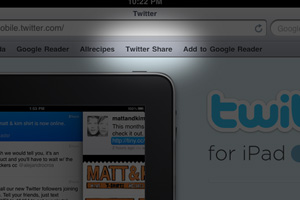It is surprisingly difficult to do this share a page link from iPad Safari to Twitter. The best solution seems to be the Twitter Share bookmarklet, which isn’t as simple as you might expect.

``javascript:(function(){window.twttr=window.twttr||{};var D=550,A=450,C=screen.height,B=screen.width,H=Math.round((B/2)-(D/2)),G=0,F=document,E;if(C>A){G=Math.round((C/2)-(A/2))}window.twttr.shareWin=window.open('http://twitter.com/share','','left='+H+',top='+G+',width='+D+',height='+A+',personalbar=0,toolbar=0,scrollbars=1,resizable=1');E=F.createElement('script');E.src='http://platform.twitter.com/bookmarklets/share.js?v=1';F.getElementsByTagName('head').appendChild(E)}());``